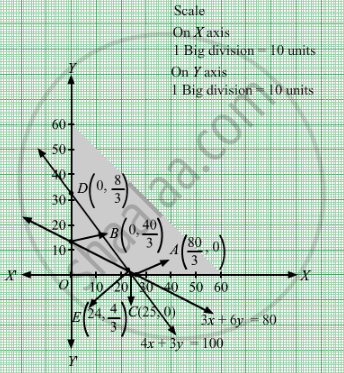# A Diet is to Contain at Least 80 Units of Vitamin a and 100 Units of Minerals. Two Foods F1 and F2 Are Available - Mathematics

Sum

A diet is to contain at least 80 units of vitamin A and 100 units of minerals. Two foods F1and F2 are available. Food F1 costs Rs 4 per unit and F2 costs Rs 6 per unit one unit of food F1 contains 3 units of vitamin A and 4 units of minerals. One unit of food F2contains 6 units of vitamin A and 3 units of minerals. Formulate this as a linear programming problem and find graphically the minimum cost for diet that consists of mixture of these foods and also meets the mineral nutritional requirements

#### Solution

Let the dietician wishes to mix units of food F1 and y units of food F2.
Clearly, $x, y \geq 0$

The given information can be tabulated as follows:

 Vitamin A Minerals Food F1 3 4 Food F2 6 3 Minimum requirement 80 100

The constraints are

$3x + 6y \geq 80$
$4x + 3y \geq 100$

It is given that cost of food F1 and F2 is Rs 4 and Rs 6 per unit respectively. Therefore, cost of units of food F1 and y units of food F2 is Rs 4x  and Rs 6y respectively.
Let Z denote the total cost
∴ Z = 4x + 6y
Thus, the mathematical formulat​ion of the given linear programmimg problem is
Minimize

$Z = 4x + 6y$ subject to

$3x + 6y \geq 80$
$4x + 3y \geq 100$

$x, y \geq 0$

First, we will convert the given inequations into equations, we obtain the following equations:
3x + 6y = 80, 4x + 3y = 100= 0 and y = 0

The line 3x + 6y = 80 meets the coordinate axis at

$A\left( \frac{80}{3}, 0 \right)$ and$B\left( 0, \frac{40}{3} \right)$ Join these points to obtain the line 3x + 6y = 80. Clearly, (0, 0) does not satisfies the inequation 3x + 6y ≥ 80. So, the region in xy-plane that does not contains the origin represents the solution set of the given equation.
The line 4x + 3y = 100 meets the coordinate axis at C(25, 0) and $D\left( 0, \frac{100}{3} \right)$  Join these points to obtain the line 4x + 3y = 100. Clearly, (0, 0) does not satisfies the inequation 4x + 3y ≥ 100. So, the region in xy-plane that does not contains the origin represents the solution set of the given equation.

Region represented by x ≥ 0 and y ≥ 0:
Since, every point in the first quadrant satisfies these inequations. So, the first quadrant is the region represented by the inequations.
These lines are drawn using a suitable scale.

The feasible region determined by the system of constraints isThe corner points are D

$\left( 0, \frac{100}{3} \right)$ , E $\left( 24, \frac{4}{3} \right)$ and $A\left( \frac{80}{3}, 0 \right)$ The values of Z at these corner points are as follows:

 Corner point Z= 4x + 6y D $\left( 0, \frac{100}{3} \right)$ 200 E $\left( 24, \frac{4}{3} \right)$ 104 $A\left( \frac{80}{3}, 0 \right)$ $\frac{320}{3}$

The minimum value of Z is Rs 104 which is at $\left( 24, \frac{4}{3} \right)$

Concept: Graphical Method of Solving Linear Programming Problems
Is there an error in this question or solution?

#### APPEARS IN

RD Sharma Class 12 Maths
Chapter 30 Linear programming
Exercise 30.3 | Q 6 | Page 39